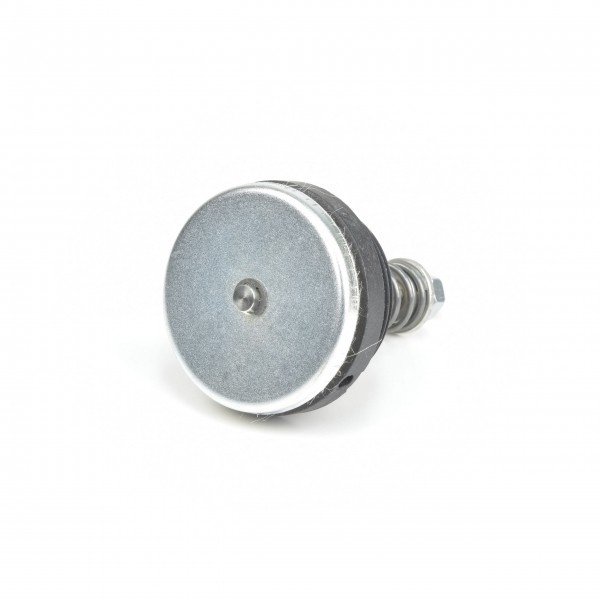# 72750206000 Integrated Relief ValvesDelivery time: 1 - 3 business days

• 72750206000
Becker Original Integrated Relief Valve 72750206000 Integrated relief valves protect... more
Product information "72750206000 Integrated Relief Valves"

Becker Original Integrated Relief Valve 72750206000
Integrated relief valves protect the pump against overstress by blowing off the excess compressed air as soon as a determined value is reached. The connection size is: G1A.

 Type of valve: Integrated Relief Valve ST-number: ST597-0-1.2 Connection size 1: G1ADT 3.10 Order number: DT3.10DT 3.10 (Index A) Order number: DT3.10-ADT 3.10 (Index B) Order number: DT3.10-BDT 3.10 (Index C) Order number: DT3.10-CDT 3.10 (Index D) Order number: DT3.10-DDT 3.10 (Index E) Order number: DT3.10-EDT 3.10 (Index F) Order number: DT3.10-FDT 3.16 Order number: DT3.16DT 3.16 (Index A) Order number: DT3.16-ADT 3.16 (Index B) Order number: DT3.16-BDT 3.16 (Index C) Order number: DT3.16-CDT 3.16 (Index D) Order number: DT3.16-DDT 3.16 (Index E) Order number: DT3.16-EDT 3.16 (Index F) Order number: DT3.16-FDT 3.16 (Index G) Order number: DT3.16-GDT 3.16 DTK Order number: DT3.16DTKDT 3.25 Order number: DT3.25DT 3.25 (Index A) Order number: DT3.25-ADT 3.25 (Index B) Order number: DT3.25-BDT 3.25 (Index C) Order number: DT3.25-CDT 3.25 (Index D) Order number: DT3.25-DDT 3.25 (Index E) Order number: DT3.25-EDT 3.25 (Index F) Order number: DT3.25-FDT 3.25 (Index G) Order number: DT3.25-GDT 3.25 DTK Order number: DT3.25DTKDT 3.25 K (Index A) Order number: DT3.25K-ADT 3.25 K (Index B) Order number: DT3.25K-BDT 3.25 K (Index D) Order number: DT3.25K-DDT 3.25 K (Index E) Order number: DT3.25K-EDT 3.25 K (Index F) Order number: DT3.25K-FDT 3.25 K (Index G) Order number: DT3.25K-GDT 3.40 Order number: DT3.40DT 3.40 (Index A) Order number: DT3.40-ADT 3.40 (Index B) Order number: DT3.40-BDT 3.40 (Index C) Order number: DT3.40-CDT 3.40 (Index D) Order number: DT3.40-DDT 3.40 (Index E) Order number: DT3.40-EDT 3.40 (Index F) Order number: DT3.40-FDT 3.40 (Index G) Order number: DT3.40-GDT 3.40 K Order number: DT3.40KDT 3.40 K (Index A) Order number: DT3.40K-ADT 3.40 K (Index B) Order number: DT3.40K-BDT 3.40 K (Index D) Order number: DT3.40K-DDT 3.40 K (Index E) Order number: DT3.40K-EDT 3.40 K (Index F) Order number: DT3.40K-FDT 3.40 K (Index G) Order number: DT3.40K-GDT 3.6 Order number: DT3.6DT 3.6/08 Order number: DT3.608EP100/P100i Order number: EP100P100iEP40/P40i (Index A) Order number: EP40P40i-AEPV100/P100i Order number: EPV100P100i EPV100/PV100i Order number: EPV100PV100iEPV40/P40i (Index A) Order number: EPV40P40i-A EPV40/PV40i (Index A) Order number: EPV40PV40i-AEV100/P100i Order number: EV100P100iEV100/PV100i Order number: EV100PV100iEV100/V100i Order number: EV100V100iEV40/P40i (Index A) Order number: EV40P40i-AEV40/PV40i (Index A) Order number: EV40PV40i-AEV40/V40i (Index A) Order number: EV40V40i-AVT 3.10 Order number: VT3.10VT 3.10 (Index A) Order number: VT3.10-AVT 3.10 (Index B) Order number: VT3.10-BVT 3.10 (Index C) Order number: VT3.10-CVT 3.10 (Index D) Order number: VT3.10-DVT 3.10 (Index E) Order number: VT3.10-EVT 3.16 Order number: VT3.16VT 3.16 (Index A) Order number: VT3.16-AVT 3.16 (Index B) Order number: VT3.16-BVT 3.16 (Index C) Order number: VT3.16-CVT 3.16 (Index D) Order number: VT3.16-DVT 3.16 (Index E) Order number: VT3.16-EVT 3.16 (Index F) Order number: VT3.16-FVT 3.16 (Index G) Order number: VT3.16-GVT 3.25 Order number: VT3.25VT 3.25 (Index A) Order number: VT3.25-AVT 3.25 (Index B) Order number: VT3.25-BVT 3.25 (Index C) Order number: VT3.25-CVT 3.25 (Index D) Order number: VT3.25-DVT 3.25 (Index E) Order number: VT3.25-EVT 3.25 (Index F) Order number: VT3.25-FVT 3.25 (Index G) Order number: VT3.25-GVT 3.40 Order number: VT3.40VT 3.40 (Index A) Order number: VT3.40-AVT 3.40 (Index B) Order number: VT3.40-BVT 3.40 (Index C) Order number: VT3.40-CVT 3.40 (Index D) Order number: VT3.40-DVT 3.40 (Index E) Order number: VT3.40-EVT 3.40 (Index F) Order number: VT3.40-FVT 3.40 (Index G) Order number: VT3.40-G VT 3.6 Order number: VT3.6 VT 3.6/08 Order number: VT3.608 VT 3.6/08 (Index B) Order number: VT3.608-BVT 3.60 Order number: VT3.60 VT 4.10 Order number: VT4.10 VT 4.16 Order number: VT4.16 VT 4.16/0-34 Order number: VT4.160-34 VT 4.25 Order number: VT4.25 VT 4.25 (Index A) Order number: VT4.25-A VT 4.25 (Index B) Order number: VT4.25-B VT 4.25 (Index C) Order number: VT4.25-C VT 4.25/0-06 (Index B) Order number: VT4.250-06-B VT 4.40 Order number: VT4.40 VT 4.40 (Index A) Order number: VT4.40-A VT 4.40 (Index C) Order number: VT4.40-C VT 4.40/0-400 Order number: VT4.400-400VT 4.40/0-400 (Index C) Order number: VT4.400-400-C VT 4.40/0-65 (Index A) Order number: VT4.400-65-A VT 4.40/0-65 (Index C) Order number: VT4.400-65-C VT 4.40/0-90 (Index B) Order number: VT4.400-90-B VT 4.40/0-90 (Index C) Order number: VT4.400-90-CVX 3.6/08 (Index B) Order number: VX3.608-B VX 4.10 Order number: VX4.10VX 4.10/0-89 Order number: VX4.100-89 VX 4.16 Order number: VX4.16 VX 4.25 Order number: VX4.25VX 4.25 (Index C) Order number: VX4.25-CVX 4.25/0-44 Order number: VX4.250-44VX 4.25/0-44 (Index C) Order number: VX4.250-44-C VX 4.40 Order number: VX4.40VX 4.40 (Index C) Order number: VX4.40-C
Viewed